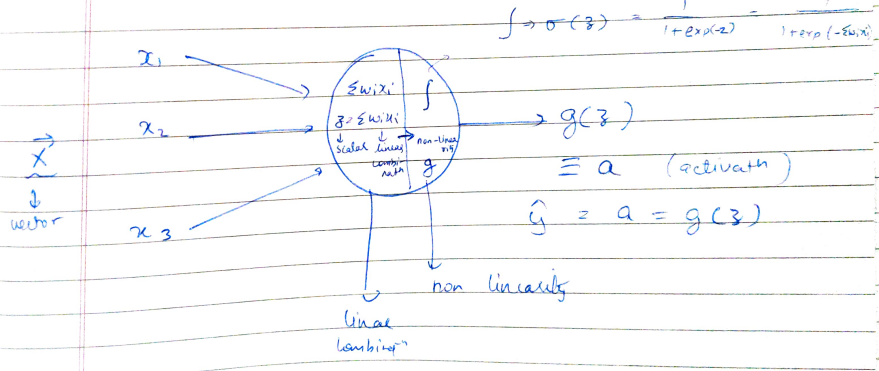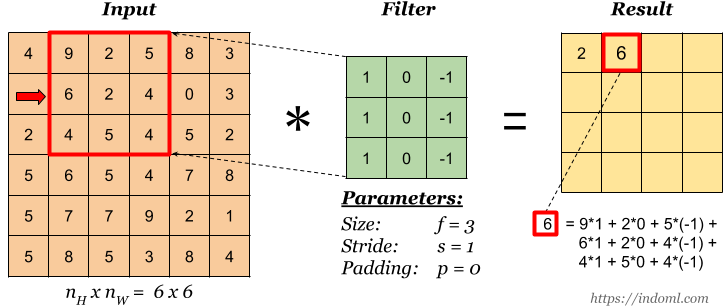# Convolutional Neural NetworksBasic fundamentals of CNN. CNN’s are a special type of ANN which accepts images as inputs. Below is the representation of a basic neuron of an ANN which takes as input X vector.

CNN’s are a special type of ANN which accepts images as inputs. Below is the representation of a basic neuron of an ANN which takes as input X vector. The values in the X vector is then multiplied by corresponding weights to form a linear combination. To thus, a non-linearity function or an activation function is imposed so as to get the final output.Neuron representation, Image by author

## Why CNN?

Talking about grayscale images, they have pixel ranges from 0 to 255 i.e. 8-bit pixel values. If the size of the image is NxM, then the size of the input vector will be NM. For RGB images, it would be NM*3. Consider an RGB image with size 30x30. This would require 2700 neurons. An RGB image of size 256x256 would require over 100000 neurons. ANN takes a vector of inputs and gives a product as a vector from another hidden layer that is fully connected to the input. The number of weights, parameters for 224x224x3 is very high. A single neuron in the output layer will have 224x224x3 weights coming into it. This would require more computation, memory, and data. CNN exploits the structure of images leading to a sparse connection between input and output neurons. Each layer performs convolution on CNN. CNN takes input as an image volume for the RGB image. Basically, an image is taken as an input and we apply kernel/filter on the image to get the output. CNN also enables parameter sharing between the output neurons which means that a feature detector (for example horizontal edge detector) that’s useful in one part of the image is probably useful in another part of the image.

## Convolutions

Every output neuron is connected to a small neighborhood in the input through a weight matrix also referred to as a kernel or a weight matrix. We can define multiple kernels for every convolution layer each giving rise to an output. Each filter is moved around the input image giving rise to a 2nd output. The outputs corresponding to each filter are stacked giving rise to an output volume.Convolution operation, Image by indoml

Here the matrix values are multiplied with corresponding values of kernel filter and then summation operation is performed to get the final output. The kernel filter slides over the input matrix in order to get the output vector. If the input matrix has dimensions of Nx and Ny, and the kernel matrix has dimensions of Fx and Fy, then the final output will have a dimension of Nx-Fx+1 and Ny-Fy+1. In CNN’s, weights represent a kernel filter. K kernel maps will provide k kernel features.

## How are deep learning, artificial intelligence and machine learning related

What is the difference between machine learning and artificial intelligence and deep learning? Supervised learning is best for classification and regressions Machine Learning models. You can read more about them in this article.

## Artificial Intelligence, Machine Learning, Deep Learning

Artificial Intelligence (AI) will and is currently taking over an important role in our lives — not necessarily through intelligent robots.

## Architecture and Training Of Convolutional Neural Networks (7 points):

This post provides the details of the architecture of Convolutional Neural Network (CNN), functions and training of each layer, ending with a summary of the training of CNN.

## Start a Career in Machine Learning and Artificial Intelligence

Enroll now at best Artificial Intelligence training in Noida, - the best Institute in India for Artificial Intelligence Online Training Course and Certification.

## AI(Artificial Intelligence): The Business Benefits of Machine Learning

Enroll now at CETPA, the best Institute in India for Artificial Intelligence Online Training Course and Certification for students & working professionals & avail 50% instant discount.